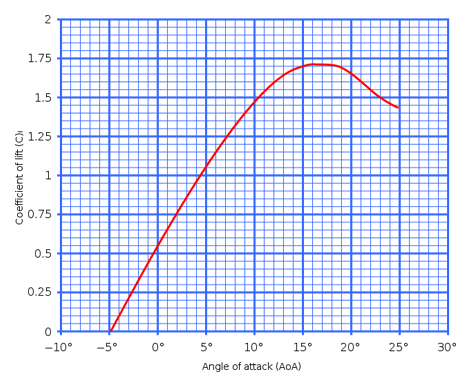Lift coefficientBy Wikipedia,
the free encyclopedia,

http://en.wikipedia.org/wiki/Lift_coefficient

The lift coefficient (CL or CZ) is a dimensionless coefficient that relates the lift generated by an airfoil, the dynamic pressure of the fluid flow around the airfoil, and the planform area of the airfoil. It may also be described as the ratio of lift pressure to dynamic pressure.

## Aircraft lift coefficient

Lift coefficient may be used to relate the total lift generated by an aircraft to the total area of the wing of the aircraft. In this application it is called the aircraft lift coefficient CL.

The lift coefficient CL is equal to:$C_L={L \over \frac{1}{2}\rho v^2A} = \frac{L}{q A}$
where

The aircraft lift coefficient can be approximated using the Lifting-line theory.

## Section lift coefficientA typical curve showing section lift coefficient versus angle of attack for a cambered airfoil.

Lift coefficient may also be used as a characteristic of a particular shape (or cross-section) of an airfoil. In this application it is called the section lift coefficient cl. It is common to show, for a particular airfoil section, the relationship between section lift coefficient and angle of attack. It is also useful to show the relationship between section lift coefficient and drag coefficient.

The section lift coefficient is based on the concept of an infinite wing of non-varying cross-section. It is not practical to define the section lift coefficient in terms of total lift and total area because they are infinitely large. Rather, the lift is defined per unit span of the wing (L'). In such a situation, the above formula becomes:$c_l={L' \over \frac{1}{2}\rho v^2c}$

where c is the chord length of the airfoil.

The section lift coefficient for a given angle of attack can be approximated using the Thin Airfoil Theory.

Note that the lift equation does not include terms for angle of attack — that is because there is no mathematical relationship between lift and angle of attack. (In contrast, there is a straight-line relationship between lift and dynamic pressure; and between lift and area.) The relationship between the lift coefficient and angle of attack is complex and can only be determined by experimentation or complex analysis. See the accompanying graph. The graph for section lift coefficient vs. angle of attack follows the same general shape for all airfoils, but the particular numbers will vary. The graph shows an almost linear increase in lift coefficient with increasing angle of attack, up to a maximum point, after which the lift coefficient falls away, although it only becomes zero at 90 degrees.. This indicates the lift coefficient at the stall of the airfoil.

The lift coefficient is a dimensionless number.

Note that in the graph here, there is still a small but positive lift coefficient with angles of attack less than zero. This is true of any airfoil with camber (asymmetrical airfoils). On a cambered airfoil at zero angle of attack the pressures on the upper surface are lower than on the lower surface.

Published in July 2009.